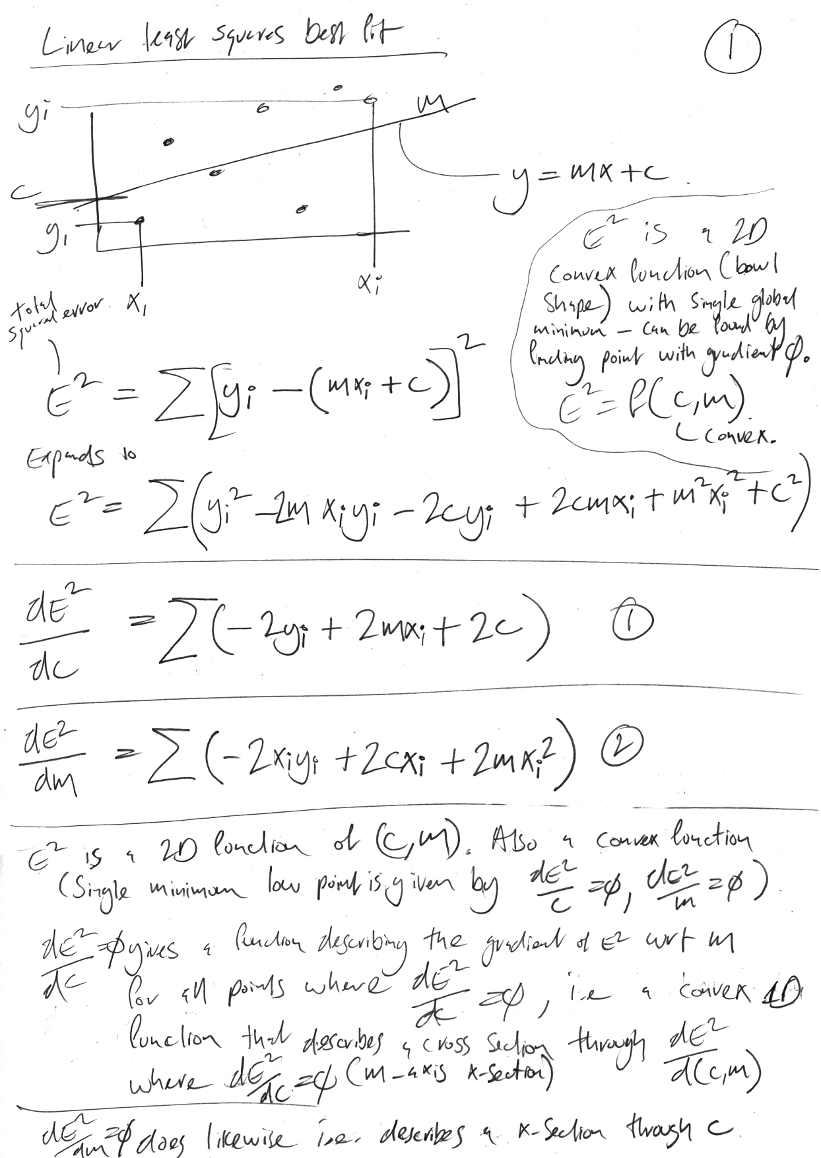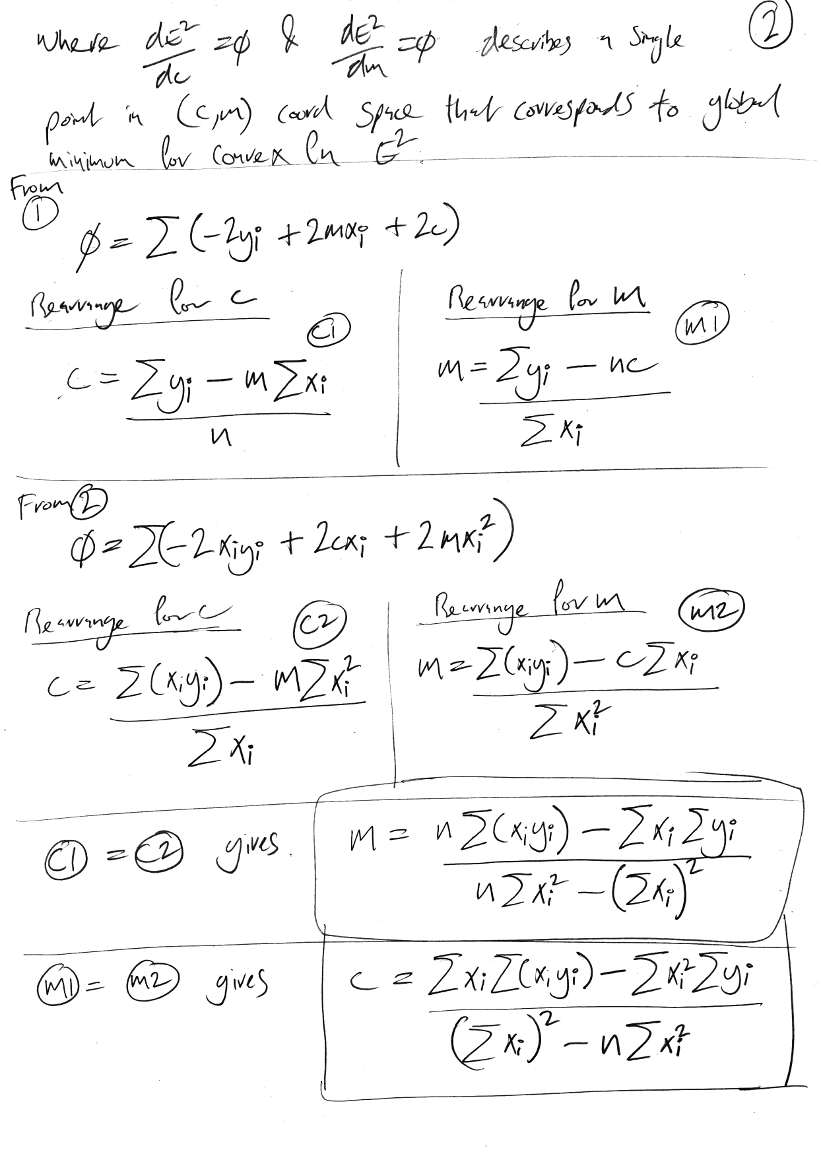﻿ Linear Least Squares

# Linear Least Squares

Task: Given a set of points in 2D euclidean space, find the line that minimises squared error. A line is described by:

$$y = mx + c$$

Therefore we wish to find the values of m and c that minimise squared error. The first step is to consider squared error as a function of m and c. I'll denote squared error with E^2.

$$E^2 = f(m, c)$$

So E^2 is a 2D surface described by the above function. Due to the nature of squared error we know that the surface happens to be a convex function (bowl shaped) with a single minimum point. Therefore we can find that mimimum point by finding the single (m,c) coordinate where the function's gradient is zero.

So we need to obtain the gradient function and solve for a gradient of zero (see derivation below). Doing so gives the following functions for m and c respectively:

$$m = \frac{n \sum_i{x_i y_i} - \sum_i{x_i} \sum_i{y_i}}{n \sum_i{x_i^2} - (\sum_i{x_i})^2} \tag{1}$$

$$c = \frac{\sum_i{x_i} \sum_i{x_i y_i} - \sum_i{x_i^2} \sum_i{y_i}}{(\sum_i{x_i})^2 - n \sum_i{x_i^2}} \tag{2}$$

When evaluating the above functions be sure to evaluate the numerator term first and check for a zero, this avoids having to evaluate the denominator and also divide by zero errors.

Colin,
October 2012

Derivation - Page 1Derivation - Page 2Copyright 2012 Colin Green.
This article is licensed under a Creative Commons Attribution 3.0 License